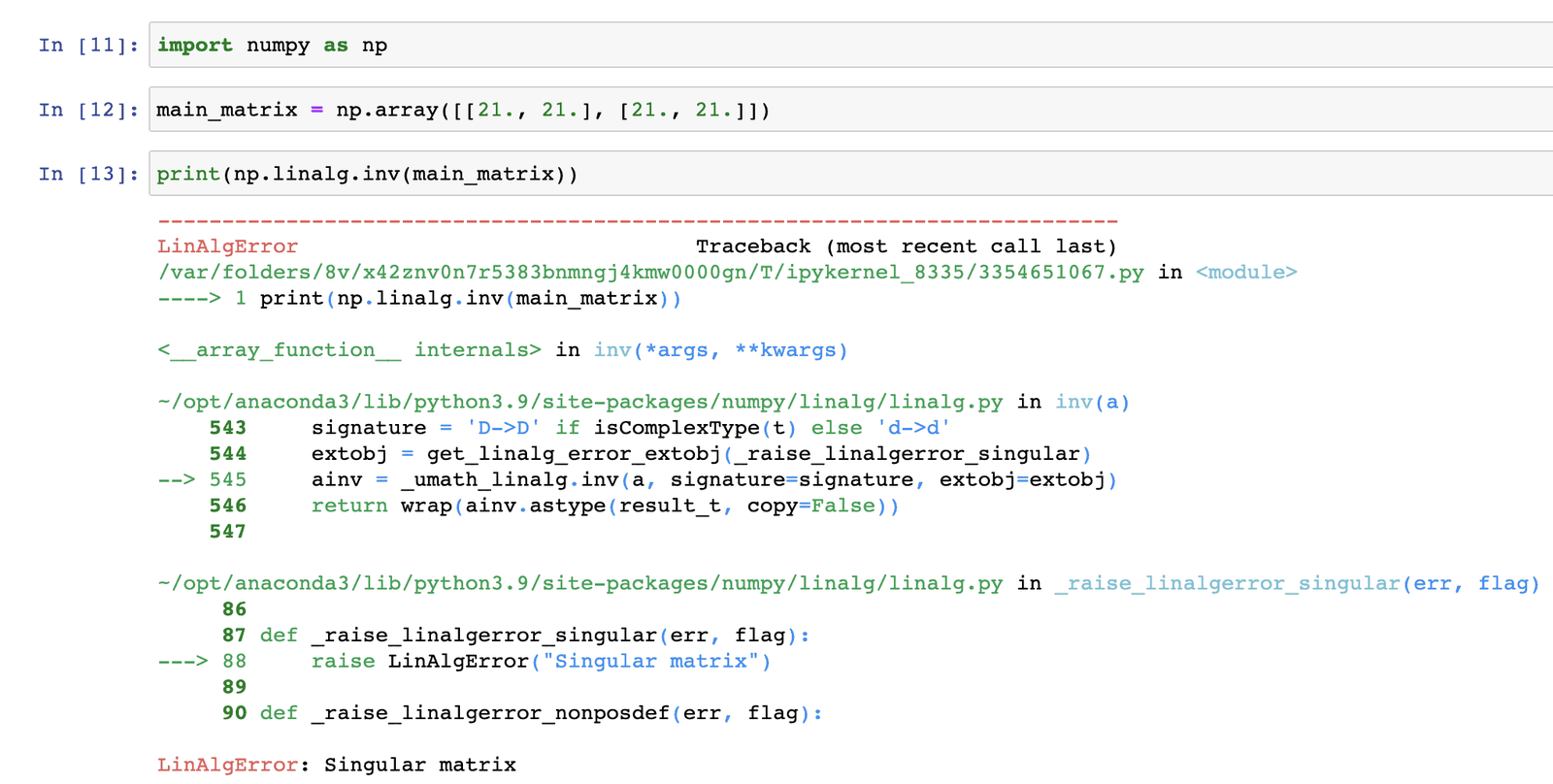# How to Fix numpy.linalg.linalgerror: singular matrix

The numpy.linalg.linalgerror: singular matrix error occurs in Python when you attempt to invert a singular matrix whose determinant is zero that cannot be inverted.

To fix the LinAlgError: Singular matrix error, create a matrix that is not singular, and the determinant is not 0.0.

### Python code that generates numpy.linalg.linalgerror: singular matrix

``````import numpy as np

#create 2x2 matrix
main_matrix = np.array([[21., 21.], [21., 21.]])

# attempt to print the inverse of matrix
print(np.linalg.inv(main_matrix))``````

OutputYou can see that we got the “LinAlgError: Singular matrix” error because the `main_matrix` is not invertible. It has the same value in all entries, making it a singular matrix with zero determinant.

The numpy.linalg.LinAlgError is an exception class for general purposes, derived from Python’s exception.

When a Linear Algebra-related condition prevents continued accurate execution of the function, this exception class is raised programmatically in linalg functions.

A matrix is invertible only if its determinant is non-zero.

If the determinant is zero, the matrix is said to be singular and has no inverse.

You can use the np.linalg.det() function from NumPy to calculate the determinant of a given matrix before you try to invert it.

``````import numpy as np

# create 2x2 matrix
main_matrix = np.array([[21., 21.], [21., 21.]])

# Printing the determinant
print(np.linalg.det(main_matrix))``````

Output

``0.0``

You can see that the determinant of the matrix is zero, which explains why we get into the error.

## How to Fix numpy.linalg.linalgerror: singular matrix error

### Code that fixes the error

``````import numpy as np

# create 2x2 matrix
main_matrix = np.array([[21., 19.], [19., 21.]])

# Printing the inverse of matrix
print(np.linalg.inv(main_matrix))``````

Output

``````[[ 0.2625 -0.2375]
[-0.2375  0.2625]]``````

You can see that the code worked without errors and will print the inverse of the main_matrix.

In this case, the main_matrix is a 2×2 matrix with a non-zero determinant, which is invertible.

The np.linalg.inv() function from the NumPy library is used to find the inverse of the main_matrix.

I hope this solution will resolve your error.

This site uses Akismet to reduce spam. Learn how your comment data is processed.